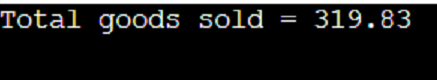# Tracing of C code

## Question

• List each line number in the program which is executed,
• After the line number describes the calculation or decision made and show the values of the variables in the expression and variables,
• For loops and if and if/else statements state whether it enters the construct and, for an if-else, whether it executes the true part of the false part,
• For print statements, show what is printed by the statement,
• If a statement is executed more than once, show it every time it is executed and the values of the variables,
• Statements that are never executed should not be included in the walkthrough.
#define _CRT_SECURE_NO_WARNINGS
#include <stdio.h>
#define MAX_ITEMS_PER_SALE 20
#define MAX_INVENTORY 10
#define MAX_SALES 20
struct Inventory
{
int sku;
int numInStock;
double price;
};
struct SaleRecord
{
int sku[MAX_ITEMS_PER_SALE];
int numEachItemSold[MAX_ITEMS_PER_SALE];
int numItemsInSale;
};

int main(void)
{
struct Inventory inventory[MAX_INVENTORY] = {
{458, 26, 21.99},
{623, 71, 12.99}
};
struct SaleRecord sales[MAX_SALES] = {
{{458}, {3}, 1},
{{458, 623}, {8,6}, 2}
};

int numInventoryItems = 2, numSales = 2;
int i, j, k, sku, numSold, found;
double sum = 0.0;
for (i = 0; i < numSales; i++)
{
for (j = 0; j < sales[i].numItemsInSale; j++)
{
sku = sales[i].sku[j];
numSold = sales[i].numEachItemSold[j];
found = 0;
for (k = 0; k < numInventoryItems && !found; k++)
{
if (inventory[k].sku == sku)
{
sum += inventory[k].price * numSold;
found = 1;
}
}
}
}
printf("Total of goods sold = %.2lf\n", sum);

return 0;
}


## Summary

In this question, we will trace the C code. According to the given code, there are two structures with the names Inventory and SaleRecord, and there are three members in each of the structures.
In the main method, some instances of both the structures have been declared and the total amount obtained on selling the good is calculated by running a nested loop.
We have explained the walkthrough to the code mentioning the line numbers.

## Explanation

We will trace the C code line by line.

Walkthrough to the code:
Line 04: We have assigned 20 to the variable “MAX_ITEMS_PER_SALE”.
Line 05: We have assigned 10 to the variable “MAX_INVENTORY”.
Line 06:We have assigned 20 to the variable “MAX_SALES”.
Line 22: the main function called

And Line 24: And in this line, we have declared the variable inventory of the Inventory structure with two instances.
Inventory = {458, 26, 21.99}
Inventory = {623, 71, 12.99}

Line 28: We have declared the variable sales of SaleRecord structure with two instances.
Sales = {{458}, {3}, 1}
Sales = {{458, 623}, {8,6}, 2}

Line 33: We have assigned value 2 to the variable “numInventoryItems”, which indicates the number of inventory items. Also, we have assigned 2 to the variable “numSales”, which indicates the number of sales records.

And Line 34: Memory allocation is done for the variables I, j, k, sku, numSold, and found.
Line 35: We have assigned the variable sum with 0.0.
Line 38: The outer for loop has started.
(i=0)
It is checked if i < numSales
0 < 2 (true)

Line 39:
Line 40: The inner for loop has started.
(j=0)
It is checked if j < sales[i].numItemsInSale
0 < 1 (true)

Line 41:
Line 42: We have assigned the variable “sku” with value sales[i].sku[j].
sku = sales.sku = 458

Line 43: numSold = sales.numEachItemSold = 3
Line 44: found = 0
Line 45:
(k=0)
0 < 2 (true) and !found = 1 (true)

Line 46: inventory.sku == sku
458 == 458 (true)

Line 48:
Line 49:
sum = sum + inventory.price * 3 = 0 + (21.99 * 3) = 65.97
Line 50: found =1
Line 45:
(k=1)
!found = 0 (false)

Line 40:
(j=1)
1 < sales.numItemsInSale (1<1) (false)

Line 38:
(i=1)
i < numSales (1 < 2) (true)

Line 40:
(j=0)
j < sales.numItemsInSale (0<2) (true)

Line 42: sku = sales.sku = 458
Line 43: numSold = sales.numEachItemSold = 8
Line 44: found=0
Line 45:
(k=0)
!found =1 (true) and (0 < 2) (true)

Line 47: inventory.sku == sku
458 == 458 (true)

Line 49: sum = sum + inventory.price * 8 = 65.97 + (21.99 * 8) = 241.89

And Line 50: found =1
Line 45:
(k=1)
!found =0 (false)

Line 40:
(j=1)
(1 < 2) (true)

Line 42: sku = sales.sku = 623
Line 43: numSold = sales.numEachItemSold = 6
Line 44: found =0
Line 45:
(k=0)
0<2 (true) and !found =1 (true)

Line 47: inventory.sku == sku
458 == 623 (false)

Line 45:
(k=1)
1<2 (true) !found=1 (true)

Line 47: inventory.sku == sku
623 == 623 (true)

Line 49: sum= sum + inventory.price * numSold = 241.89 + (12.99*6) = 319.83

Line 50: found =1

And Line 45: (k=2)
2<2 (false)

Line 38:
(i=2)
(2<2) (false)

Line 55:
It prints the sum stored.
The print statement is:
Total of Goods sold = 319.83

## Output

Trace the C code output:Also read, Function Pointer in C